Courses

# Test: Chemistry- 6

## 45 Questions MCQ Test NEET Mock Test Series | Test: Chemistry- 6

Description
This mock test of Test: Chemistry- 6 for NEET helps you for every NEET entrance exam. This contains 45 Multiple Choice Questions for NEET Test: Chemistry- 6 (mcq) to study with solutions a complete question bank. The solved questions answers in this Test: Chemistry- 6 quiz give you a good mix of easy questions and tough questions. NEET students definitely take this Test: Chemistry- 6 exercise for a better result in the exam. You can find other Test: Chemistry- 6 extra questions, long questions & short questions for NEET on EduRev as well by searching above.
QUESTION: 1

Solution:
QUESTION: 2

Solution:
QUESTION: 3

### Suppose a compound contains atoms A,B and C. The oxidation number of A is+ 2, B is+ 5 and C is –2.The possible formula of the cmpound would be

Solution:
QUESTION: 4
The oxidation number of phosphorus in its compounds varies from
Solution:
QUESTION: 5
The rducing agent used in Thermit process is
Solution:
QUESTION: 6

For the redox reaction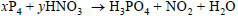Solution:
QUESTION: 7
The oxidation number of sulphur in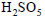is
Solution:
QUESTION: 8
The oxidation number of sulphur in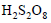is
Solution:
QUESTION: 9
A sample of 2.5 mol of hydrazine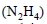loses 25 mol of electrons on being converted into a new compound X. Assuming that all of the nitrogen
appears in the new compound, what is the oxidation state of nitrogen in compound X?
Solution:
QUESTION: 10
The equivalent weight of nitrogen in the reaction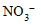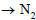is
Solution:
QUESTION: 11

Which of the following ions will undergo disproportionation reaction?

Solution:
QUESTION: 12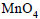is a good oxidizing agent in different media where it changes to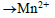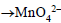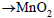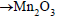The oxidatino number of Mn correspondingly reduces by
Solution:
QUESTION: 13
Coordination theory was forumulated by
Solution:
QUESTION: 14
Which of the following is a double salt ?
Solution:
QUESTION: 15
The IUPAC name of the complex compound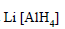is
Solution:
QUESTION: 16
The IUPAC name for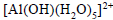is
Solution:
QUESTION: 17
The IUPAC name for the coordination compound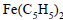is
Solution:
QUESTION: 18
Which of the following complex compounds exhibts cis-trans isomerism ?
Solution:
QUESTION: 19
The hyridization states of the central atom in the complex ions are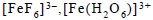and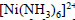are
Solution:
QUESTION: 20
Which of the following statements is correct ?
Solution:
QUESTION: 21
Consider the following spatial arrangements of the octahedral complex ion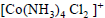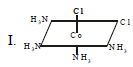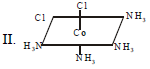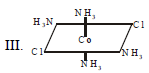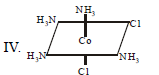Which of the following statement is incorrect regarding these stuructures ?
Solution:
QUESTION: 22
Which of the following statements is correct regarding the chirality (optical isomerism )of the cis and trans isomers of the type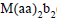(M stands for
a metal, a and b are achiral ligands and aa is a bidentate ligand) ?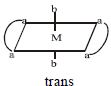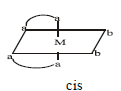Solution:
QUESTION: 23
Which of the following mixtures is known as Ziegler–Natta cAtalyst ?
Solution:
QUESTION: 24
One mole of the complex compound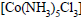gives 3 moles of ions on dissolution in water. One mole of the same complex reacts with two moles of AgNO3 solution to yield two moles of AgCl(s). The structure of the complex is
Solution:
QUESTION: 25
Which of the following have the general formula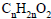?
Solution:
QUESTION: 26
Which of the following compounds will give propanoic acid as the sole product on oxidation with chromic acid
Solution:
QUESTION: 27
Trichloromethane on heating with aqueous KOH and sobsequent treatment with dilute HCl gives
Solution:
QUESTION: 28
Which of the following compounds on heating at about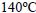gives acetic acid ?
Solution:
QUESTION: 29
In which of the following reactions does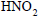act as oxidizing agent ?
Solution:
QUESTION: 30
Consider the following sequence of reactions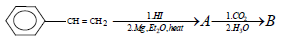The end product (B) is
Solution:
QUESTION: 31
Which of the following is the strongest acid ?
Solution:
QUESTION: 32
Which of the following can be decarboxylated most easily?
Solution:
QUESTION: 33
The general formula of alkanamides is
Solution:
QUESTION: 34
The corect order of decreasing reactivities of the following compounds towards hydrolysis under identical conditin is
Solution:
QUESTION: 35
The correct arrangement of the following compounds in the order of their decreasing boiling points is
Solution:
QUESTION: 36
The nucleophilic acyl substitution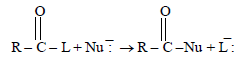will occur smoothly if
Solution:
QUESTION: 37
Which of the following compounds exists as a nonresolvable racemic mixture ?
Solution:
QUESTION: 38
The total number of secondary amines (excluding stereoisomers) having the molecular formula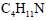is
Solution:
QUESTION: 39
The reaction of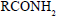with a mixture of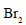and aqueous KOH gives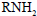as the main product. The intermediate(s) involved in this reaction is (are)
Solution:
QUESTION: 40
The end product (B) formed in the reaction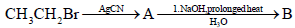Solution:
QUESTION: 41
Amongst the following the most basic compound is
Solution:
QUESTION: 42
The element that has the least tendency to show the inert-pair effect is
Solution:
QUESTION: 43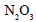is
Solution:
QUESTION: 44
The structure of oelum is
Solution:
QUESTION: 45
Which of the following bonds has the highest energy ?
Solution: